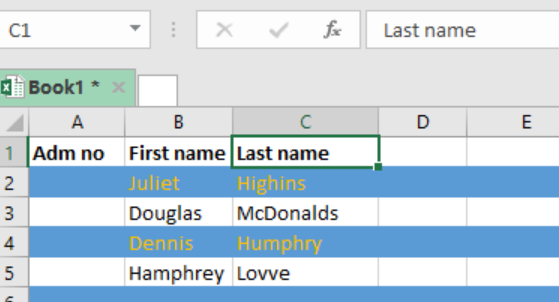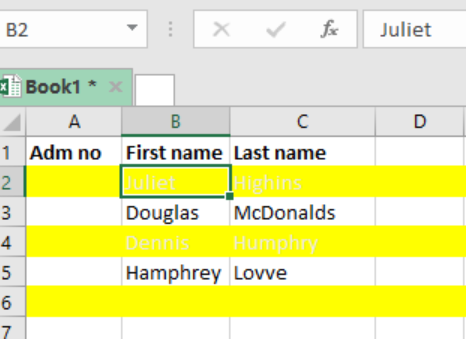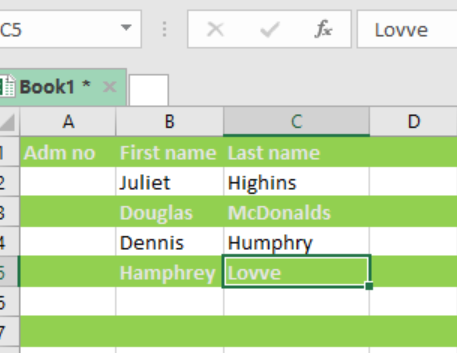Get instant live expert help with Excel or Google Sheets“My Excelchat expert helped me in less than 20 minutes, saving me what would have been 5 hours of work!”

#### Post your problem and you'll get expert help in seconds

Your message must be at least 40 characters
Our professional experts are available now. Your privacy is guaranteed.

# How to Highlight Every Other Row in Excel

While working with excel spreadsheets, there might come a time when we want to highlight every other row, which is also called zebra stripping, with conditional formatting. To do this, we can use a formula that utilizes either the ISEVEN or ISODD function. This post will guide you on how to use the functions to highlight every other row in excel.Figure 1: Highlighting every other row in excel

## General syntax of the formula

`=ISEVEN(ROW())`

or

`=ISODD(ROW())`

## Understanding the formula

This formula is fundamental in helping us highlight every other row with conditional formatting in excel. Note that the formula we use for conditional formatting will be evaluated for every cell in the selected range.

• Here, we shall run the ISEVEN and ROW functions to highlight every other row.
• The ROW function will return the number of the cell.
• The ISEVEN function will then return TRUE if the row number is even or FALSE if the row number is odd.
• The specified rule will then trigger the TRUE, and thus shade all even rows.

## What if you want to shade odd rows?

Shading odd rows is pretty much the same as shading the even rows. However, in this case we shall use the ISODD function.

## Example 1Figure 2: Highlighting every other row with ISEVEN function

In this example, we want to highlight every other row using the ISEVEN function. To do this, proceed as follows:

Step 1: Select the sheet you want to highlight every other row

Step 2: Apply conditional formatting, and set the rule as `=ISEVEN(ROW())`

Step 3: Press ok to apply the rule.

Step 4: Run the rule.

## Example 2Figure 3: Using ISODD to highlight every other row

Here, we want to highlight every other odd row. The procedure is same as that for the ISEVEN function, only that we replace ISEVEN is ISODD.

## Instant Connection to an Expert through our Excelchat Service

Most of the time, the problem you will need to solve will be more complex than a simple application of a formula or function. If you want to save hours of research and frustration, try our live Excelchat service! Our Excel Experts are available 24/7 to answer any Excel question you may have. We guarantee a connection within 30 seconds and a customized solution within 20 minutes.

Are you still looking for help with Conditional Formatting? View our comprehensive round-up of Conditional Formatting tutorials here.

### Did this post not answer your question? Get a solution from connecting with the expert.Another blog reader asked this question today on Excelchat:## Subscribe to Excelchat.coAnother blog reader asked this question today on Excelchat: